# rational

Perform rational fitting to complex frequency-dependent data

Since R2020a. Recommended over `rationalfit`.

## Description

Use the `rational` object and an interpolative algorithm to create a rational fit to frequency-dependent data.

The fit is given by the equation:

## Creation

### Syntax

``fit = rational(freq,data)``
``fit = rational(s)``
``[fit,error] = rational(___)``
``fit = rational(___,Name=Value)``

### Description

````fit = rational(freq,data)` returns a rational object with complex frequencies using the given frequency vector and network parameter data.```

example

````fit = rational(s)` returns a rational object for N-port S-parameters.```
````[fit,error] = rational(___)` also returns the error of the fit. Use any of input argument combinations from the previous syntaxes.```

example

````fit = rational(___,Name=Value)` specifies options to control aspects of fit or sets properties. You can set the NumPoles, Poles, Residues, and DirectTerm properties (since R2023a) using name-value arguments. For example, `fit = rational(s,MaxPoles=1002)` sets the maximum number of poles for the fit. Specify name-value arguments after any of the input arguments from the previous syntaxes.```

### Input Arguments

expand all

Nonnegative frequencies, specified as a vector of nonnegative frequencies in Hz.

Data Types: `double`

Network parameter data, specified as a vector, a 2-D array or a 3-D array. The length of the data values must equal the length of the frequency values.

N-port S-parameters, specified as an N-by-N matrix of elements of S sharing identical poles.

Name-Value Arguments

Specify optional pairs of arguments as `Name1=Value1,...,NameN=ValueN`, where `Name` is the argument name and `Value` is the corresponding value. Name-value arguments must appear after other arguments, but the order of the pairs does not matter.

Before R2021a, use commas to separate each name and value, and enclose `Name` in quotes.

Example: `TendsToZero = true` | `'TendsToZero',true`

Relative error tolerance, specified as a scalar less than or equal to zero in dB.

Example: `Tolerance=-30`

Data Types: `double`

Behavior of fit for large S-parameters, specified as `1` (`true`) or `0` (`false`). When `true`, the direct term in the fit is set to zero so that the rational fit `F(S)` tends to zero as `S` approaches infinity. When `false`, a nonzero direct term is allowed.

Example: `TendsToZero=true`

Data Types: `logical`

Maximum number of poles, specified as a scalar nonnegative integer.

Example: `MaxPoles=1002`

Data Types: `double`

Error metrics in the `rational` object, specified as one of the following:

• If you specify `'ErrorMetric'` as `'default'`, the `rational` object distributes the error evenly.

• If you specify `'ErrorMetric'` as `'Relative'`, the `rational` object fits both peaks and valleys or gets smaller error for smaller values.

Example: `ErrorMetric='Relative'`

Data Types: `char`

Ignores low-level noise in the data, specified as a scalar.

Example: `NoiseFloor=-60`

Data Types: `double`

Pole stability, specified as a logical `true` (`1`) or `false` (`0`). When you specify true, all the poles of the fit are stable. When you specify false,the poles can be anywhere in the complex plane.

Example: `Causal=false`

Maximum value of the quality factor of the poles of the fit, specified as a positive scalar.

Example: `Qlimit=1100`

Data Types: `double`

Data reduction, specified as a logical `1` (`true`) or `0` (`false`). When you specify true, the function reduces the data for the fitter to save memory and computation time. Reduce data for the fitter in order to save memory and computation time, specified as a logical `true` or `false`.

Example: `ColumnReduce=false`

Display options for the fitting algorithm of the rational object, specified as one of the following:

• `'off'`— No display

• `'on'`— Printed information

• `'plot'`— Plots of the interpolation progress

• `'both'`— Both printed information and plots.

Example: `Display='on'`

Data Types: `char`

## Properties

expand all

Number of ports in the original S-parameter data, returned as a scalar integer. The value of the `NumPorts` is determined from the S-parameter data.

Number or length of poles in the fit, specified as a nonegative integer (since R2023a). If you do not specify the `NumPoles` property, then the object sets the property automatically.

Example: `10`

Poles of the fit or complex values of A in the equation provided in the description, specified as a row vector of length `NumPoles`-by-`1`. When you specify this property, the object sets the Residues, DirectTerm, and ErrDB properties or you can set them separately (since R2023a).

If you do not specify the `Poles` property, then the object sets the property automatically.

Example: ```[-5.4e+10 + 4.7e+10i;-5.4e+10 - 4.7e+10i]``` specifies poles for a two-pole fit

Data Types: `double`

Residues in the fit or complex values of C in the equation provided in the description, specified as a 3-D matrix. The first two dimension of `Residues` must be of the same length as the first two dimension of `data` and the last dimension of `Residues` must be of the same length as `Poles`. If you specify this property, the object sets the DirectTerm and ErrDB properties or you can set them separately (since R2023a).

If you do not specify the `Residues` property, then the object sets the property automatically as a 3-D matrix of length `NumPorts`-by-`NumPorts`-by-`NumPoles`.

Data Types: `double`

Direct term in the fit or the value of D in the equation provided in the description, specified as a 2-D matrix. The length of this matrix must be the same as the first two dimensions of the `data`. If you specify this property, the object sets the ErrDB property (since R2023a).

Note

You must specify this argument with `Poles` and `Residues`.

If you do not specify this property, then the object sets the property automatically as a t2-D matrix of length `NumPorts`-by-`NumPorts`.

Example: `[0 0;0 0]`

Data Types: `double`

Error between the fit and the original data, returned as a scalar in dB.

## Object Functions

 `timeresp` Time response for rational objects `stepresp` Step-signal response for rational object and `rationalfit` function object `freqresp` Frequency response of rational object and `rationalfit` function object `pwlresp` Calculate time response of piecewise linear input signal `impulse` Impulse response for rational function object `ispassive` Return true if rational fit output is passive at all frequencies `makepassive` Enforce passivity of rational fit `passivity` Plot passivity of N-by-N rational fit output `generateSPICE` Generate SPICE file from `rationalfit` of S-parameters `abcd` Construct state-space matrices from `rational` object `zpk` Compute zeros, poles, and gain of rational object

## Examples

collapse all

Create S-Parameters from the file named `passive.s2p`.

`S = sparameters('passive.s2p');`

Perform rational fitting of the S-parameters.

`fit = rational(S);`

Create an S-Parameters object from the file named `default.s2p`. Perform rational fitting of the S-Parameters.

```S = sparameters('default.s2p'); fit = rational(S,Display='plot')```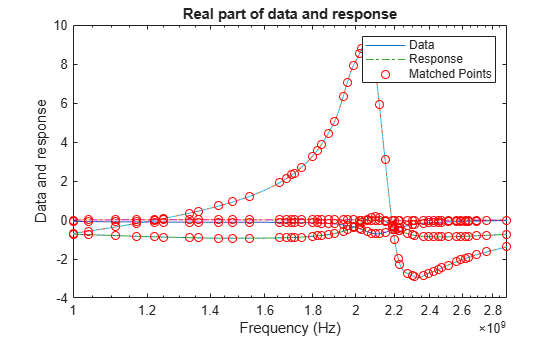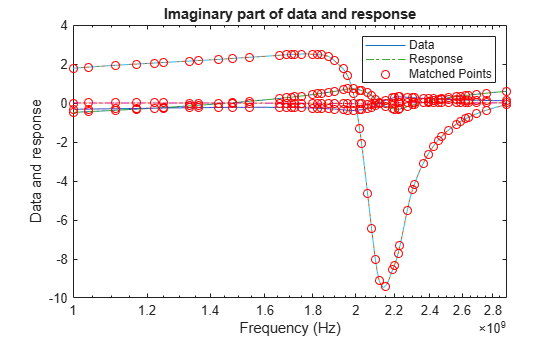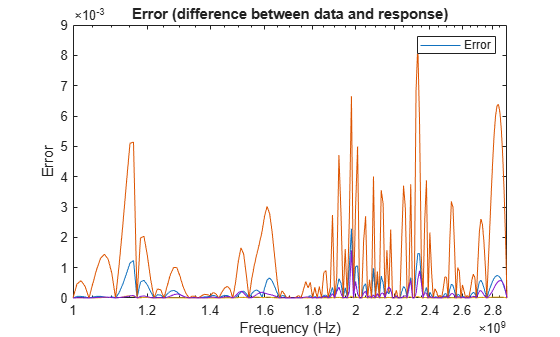```fit = rational with properties: NumPorts: 2 NumPoles: 52 Poles: [52x1 double] Residues: [2x2x52 double] DirectTerm: [2x2 double] ErrDB: -22.6872 ```

Calculate the zeros, poles, gain, and DC gain of the rational object.

`[z,p,k,dcgain] = zpk(fit)`
```z=2×2 cell array {51x1 double} {51x1 double} {51x1 double} {51x1 double} ```
```p=2×2 cell array {52x1 double} {52x1 double} {52x1 double} {52x1 double} ```
```k = 2×2 1010 × 1.0544 -0.0194 0.9158 0.0377 ```
```dcgain = 2×2 0.1289 -0.0838 -0.1209 0.7649 ```

Create an S-parameters object from the specified S2P file.

```S = sparameters('sawfilterpassive.s2p'); f = S.Frequencies;```

Create a `rational` object with the tolerance of `-`40 dB.

`fit = rational(S,Tolerance=-40);`

Compare the fit to the data. You can see the deviations at the smaller values on a semi-log plot.

```dresp = freqresp(fit,f); plot(f,20*log10(abs(squeeze(S.Parameters(2,1,:)))),... f,20*log10(abs(squeeze(dresp(2,1,:)))),f,20*log10(abs(squeeze(S.Parameters(2,1,:)-dresp(2,1,:))))) title('Default Fitting for sawfilterpassive.s2p'); ylabel('dB'); xlabel('Frequency (Hz)'); legend('Data','Fit','Error','Location','northwest');```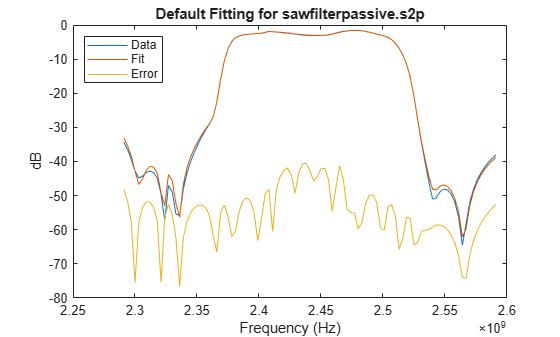Create a `rational` object with `'ErrorMetric'` set to `'Relative'` to fit both peaks and valleys.

`rfit = rational(S,Tolerance=-40,ErrorMetric='Relative');`

Compare the fit to the data. The peaks and valleys are fitted.

```rresp = freqresp(rfit,f); figure(2) plot(f,20*log10(abs(squeeze(S.Parameters(2,1,:)))),... f,20*log10(abs(squeeze(rresp(2,1,:)))), f, 20*log10(abs(squeeze(S.Parameters(2,1,:)-rresp(2,1,:))))); title('Relative Error Fitting for sawfilterpassive.s2p'); ylabel('dB'); xlabel('Frequency (Hz)'); legend('Data','Fit','Error','Location','northwest');```Create an S-parameters object from the specified S2P file.

```S = sparameters('passive.s2p'); f = S.Frequencies; data = S.Parameters;```

Set one of the data entries to zero.

`data(2,2,:) = 0;`

Create a `rational` object with the tolerance of `-40` dB.

`fit = rational(f,data,Tolerance=-40);`

Compare the fit to the data. The fit and the data match closely.

```xresp = freqresp(fit,f); figure(3) plot(f,abs(squeeze(data(2,1,:))),f,abs(squeeze(xresp(2,1,:))))```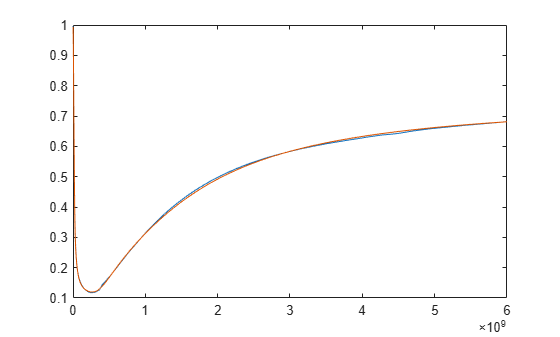Add noise to the data and create a `rational` object.

```rng(1); noisyData = data + 1e-4 * rand(size(S.Parameters)); nfit = rational(f,noisyData,Tolerance=-40);```

Compare the fit to the data with noise. Noise cannot be fitted because this is a data with a higher order fit with a worse error metric.

```nresp = freqresp(nfit,f); figure(4) plot(f,abs(squeeze(noisyData(2,2,:))),f,abs(squeeze(nresp(2,2,:))))```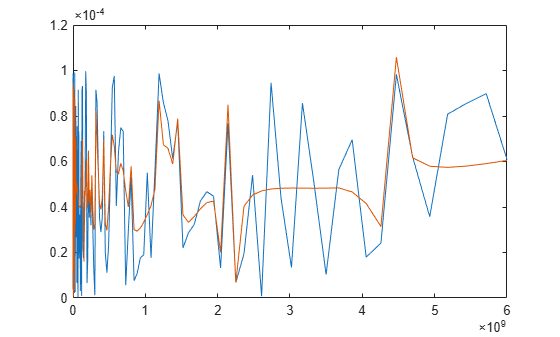Create a `rational` object with the noise floor of `-60` dB and plot the fit. The fitter ignores low-level noise.

```ffit = rational(f,noisyData,Tolerance=-40,NoiseFloor=-60); fresp = freqresp(ffit,f); figure(5) plot(f,abs(squeeze(noisyData(2,2,:))),f,abs(squeeze(fresp(2,2,:))))```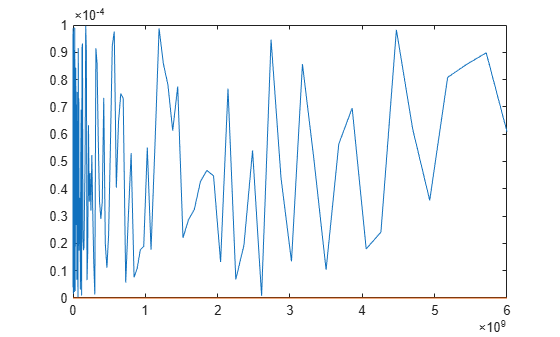Since R2023a

Create an S-parameter object.

`S = sparameters('default.s2p');`

Perform a default fit.

`fit = rational(S)`
```fit = rational with properties: NumPorts: 2 NumPoles: 52 Poles: [52x1 double] Residues: [2x2x52 double] DirectTerm: [2x2 double] ErrDB: -22.6872 ```

Limit the number of poles to four, and note that and that the error in `fit1` is higher than the error in `fit`.

`fit1 = rational(S,NumPoles=4)`
```fit1 = rational with properties: NumPorts: 2 NumPoles: 4 Poles: [4x1 double] Residues: [2x2x4 double] DirectTerm: [2x2 double] ErrDB: -14.7945 ```

Force the rational object to use twice the number of poles in `fit`. Note that the error in `fit2` is lower than `fit1`.

`fit2 = rational(S,Poles=2*fit.Poles)`
```fit2 = rational with properties: NumPorts: 2 NumPoles: 52 Poles: [52x1 double] Residues: [2x2x52 double] DirectTerm: [2x2 double] ErrDB: -18.7258 ```

 Nakatsukasa, Yuji, Olivier Sète, and Lloyd N. Trefethen. “The AAA Algorithm for Rational Approximation.” SIAM Journal on Scientific Computing 40, no. 3 (January 2018): A1494–1522. https://doi.org/10.1137/16M1106122.